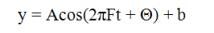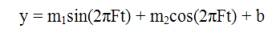U.S. Department of Transportation
1200 New Jersey Avenue, SE
Washington, DC 20590
202-366-4000

Federal Highway Administration Research and Technology
Coordinating, Developing, and Delivering Highway Transportation InnovationsThis report is an archived publication and may contain dated technical, contact, and link information
 Federal Highway Administration > Publications > Research Publications > LTPP Publications > 02034 > Long-Term Pavement Performance Materials Characterization Program: Verification of Dynamic Test Systems With An Emphasis On Resilient Modulus
Publication Number: FHWA-RD-02-034
Date: September 2005

# Long-Term Pavement Performance Materials Characterization Program: Verification of Dynamic Test Systems With An Emphasis On Resilient Modulus

## APPENDIX B. DETERMINATION OF PHASE ANGLE

This appendix provides one method for calculating phase angles for the sinusoidal dynamic response checks performed as part of this procedure. Other methods may be used to perform this calculation; this procedure is included here only as an example and not necessarily as the preferred or "best" approach. This calculation is based upon the LINEST function utilized in Microsoft® Excel.

### THEORY

The sinusoidal frequency and phase angle response tests produce time-history data for each data channel. The data should have four columns: time, load, LVDT #1, and LVDT #2. It is desired to derive from these data the phase angle between load and LVDT #1 and load and LVDT #2. A linear regression algorithm using the method of least squares can produce amplitude and phase data if given an estimate for frequency.

The reference (load) and channel (LVDT #1 or LVDT #2) data are in the form of:(2)

where

A = amplitude
cos = cosine
F = the frequency
t = time
T = phase shift with respect to the time data
b = offset

The equation shown above can be rearranged as follows:(3)

where

A = square root of (m12 + m22)

and T = -arctan(m1/m2)

let x1 = sin(2pFt)

let x2 = cos(2pFt), therefore,

y = m1x1 +m2x2 + b.

### PROCEDURE

LINEST uses the least squares method to calculate a straight line that best fits the data and returns an array that describes the line. With a column in Excel generated for x1 and x2, the LINEST function can be utilized given an estimate for F. It will return an array with m2, m1, and b. 1 In addition, it will return the R2 value. 2 Using the m2, m1, and b coefficients to describe the properties of the load and displacement data, the phase angle between the channels can be determined. It has been found that this procedure works best with only a few cycles of data.

In Excel, import the raw data file acquired from the DAS for the given test. For this example, it is assumed that time will be in column A, load in column B, LVDT #1 in column C, and LVDT #2 in column D, and that the first row is header information and that the data start in row 2. Insert six columns between the load and LVDT #1 and four columns between LVDT #1 and LVDT #2. LVDT #1 should now be in column I and LVDT #2 should be in column N.

Label column C “sine” and perform the following calculation in cell C2: SIN(2*PI()*\$H\$2*A2). Copy this calculation for all values of time. Similarly, label column D “cosine” and perform the following calculation in cell D2: COS(2*PI()*\$H\$2*A2). Copy this calculation for all values of time.

Label column E “m2cosine,” column F “m1sine,” column G “b-offset,” and column H “Frequency.” In cell H2, input the frequency for the particular test under analysis. Select block E2:G6. Type the formula “=LINEST(B2:B502, C2:D502, TRUE, TRUE)” and press ENTER while holding the CONTROL and SHIFT keys down. In this example formula, B2:B502 equals the known y’s and C2:D502 equals the known x’s. Please note that this is only an example formula. The true range for known x’s and known y’s must be input in the formula.

In cell E10, perform the calculation (-ATAN(F2/E2))*180/PI(). In cell F10, perform the calculation SQRT((E2*E2)+(F2*F2)). Label cell E9 “phase-load” and cell F9 “amp-load.”

Proceed to cell J2 and select block J2:L6. Input the LINEST formula again as previously discussed. Input the range of load values for “known y’s” (example—I2:I502), the range of values in columns C and D under “known x’s” (example—C2:D502), and input “TRUE” for “const” and “stats.” This will return a similar matrix as before.

In cell J10, perform the calculation (-ATAN(K2/J2))*180/PI(). In cell K10 perform the calculation SQRT((J2*J2)+(K2*K2)). Label cell J9 “phase-LVDT1” and cell K9 “amp-LVDT1.”

In cell J15, perform the calculation J10-E10 to determine the Phase Difference (degrees) between LVDT #1 and the load. In cell K15, perform the calculation ABS((J10-E10/360)*(1/\$H\$2)) to determine the Time Delay(s) between LVDT #1 and the load.

Perform the same sequence of calculations for all deformation channels.

1. For a detailed description of the LINEST function, go to the Excel “Help” dialog box and search for “LINEST.”
2. If the frequency is embedded as a variable in the sin and cos column data, it can be varied to optimize R2.